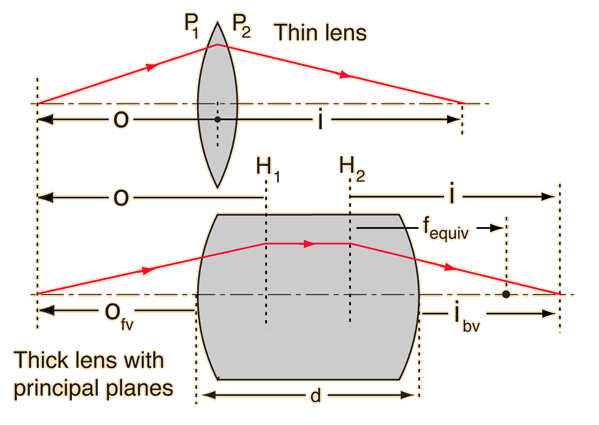# Principal Planes

The principal planes are two hypothetical planes in a lens system at which all the refraction can be considered to happen. They are typically denoted by H1 and H2. For a given set of lenses and separations, the principal planes are fixed and do not depend upon the object position. The thin lens equation can be used, but it leaves out the distance between the principal planes. The focal length f is that given by Gullstrand's equation. The principal planes for a thick lens are illustrated. For practical applications, it is often useful to use the front and back vertex powers.A thick lens can be characterized by its surface powers, its index of refraction, and its thickness.

For glass with index of refraction nlens =

the front surface power for lens radius R1 = m is P1 = m-1

and the back surface power for lens radius R2 = m is P2 = m-1

(Note that for a double convex lens, the front surface radius R1 is positive and the back surface radius R2 is negative according to the Cartesian sign convention.)

A thin lens would have approximate power Pthin lens = P1 + P2 = m-1.

The thin lens focal length would be f = m = cm.

A thick lens of thickness d = cm = m would have effective power given by Gullstrand's equation:

Pthick lens = m-1

The equivalent focal length for the thick lens would be fequiv = m = cm.

For the thin lens, we can calculate the image distance. For object distance

o = m, the image distance is

i = m.

Remember that an object in front of the lens gives a negative object distance. Also remember that an object inside the focal length does not form a real image. A negative image distance impies a virtual image.

Now for the thick lens, we will assume that the object is the same distance in front of the lens, so that the object distance from the front vertex is

ofv = m.

To use the lens equation for the thick lens, we must find the object distance to the first principal plane H1, so we must calculate the distance from the front vertex to that plane.

Front vertex to H1 = h1 = m so othick lens = m.

The lens equation, using Pthick lens, then gives the image distance

ithick lens = m.

But since this image distance is from the second principal plane H2, we need the separation between the principal planes.

H2 - H1 = m.

This locates the image for us, but if we want the image distance from the back vertex, we have to calculate the distance from H2 to that vertex.

H2 to back vertex = m.

The image distance from the back vertex is then

ibv = m.

The total distance from the object to the image for the thick lens is

othick + (H2 - H1) + ithick = m,

compared to o+i = m for the thin lens.

Sometimes for thick lenses it is more useful to state the back vertex power and focal length. For this lens:

Pback vertex = m-1.

Back vertex focal length = fback vertex = m = cm.

 Image position from principal planes
Index

Lens concepts

Thick lens concepts

 HyperPhysics***** Light and Vision R Nave
Go Back